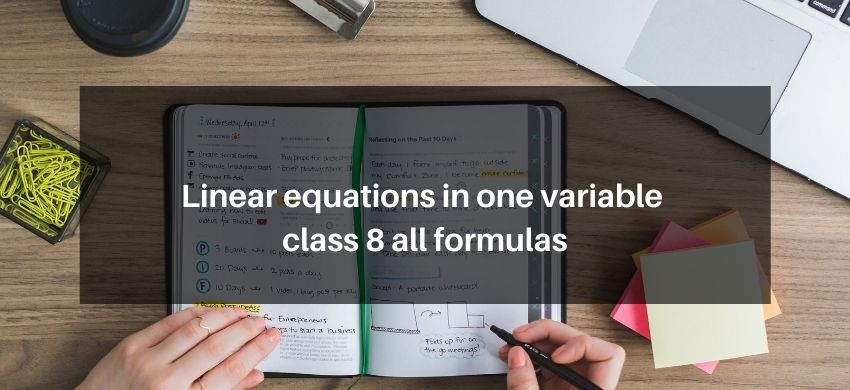# Linear equations in one variable class 8 all formulasHey, students are you looking for Linear equations in one variable class 8 all formulas? If yes. Then you are at the right place. In this post, we have listed all the formulas of Linear equation in one variable class 8 that you can use to learn and understand the concepts easily.

If you want to improve your class 8 Math, Linear equations in one variable concept, then it is super important for you to learn and understand all the formulas.

By using these formulas you will learn about what is equation, what are Linear equations in one variable, and Example of Linear equations in one variable.

With the help of these formulas, you can revise the entire chapter easily.

## Linear equations in one variable class 8 all formulas

• Equation: An equation is a statement of equality which contains one or more unknown quantity/ quantities (or variable/variables) is called an equation.
• Linear Equation in One Variable: An equation is called linear equation if it has only one degree i.s., the highest power of the variable appearing in equation is 1, and the form of linear equation is Ax + By = C here, x and y are variables, A and B are coefficients and C is a constant.
• Transposition is the process in which any term of an equation may be taken to the other side with sign changed.
• An equation which can be expressed in the form $a x+b=0$, where $a \neq 0$, is called a simple linear equation in the variable $x$.
• Thus, $a x+b=0$, where $a \neq 0$, is called the standard form of $a$ simple linear equation.